xPredominance diagramEncyclopedia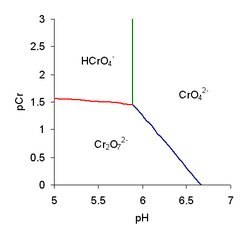A predominance diagram purports to show the conditions of concentration and pH where a chemical species
Chemical compound
A chemical compound is a pure chemical substance consisting of two or more different chemical elements that can be separated into simpler substances by chemical reactions. Chemical compounds have a unique and defined chemical structure; they consist of a fixed ratio of atoms that are held together...

has the highest concentration in solutions in which there are multiple acid-base equilibria
Chemical equilibrium
In a chemical reaction, chemical equilibrium is the state in which the concentrations of the reactants and products have not yet changed with time. It occurs only in reversible reactions, and not in irreversible reactions. Usually, this state results when the forward reaction proceeds at the same...

. The lines on a predominance diagram indicate where adjacent species have the same concentration. Either side of such a line one species or the other predominates, that is, has higher concentration relative to the other species.

To illustrate a predominance diagram, part of the one for chromate is shown at the right. pCr stands for minus the logarithm of the chromium concentration and pH
PH
In chemistry, pH is a measure of the acidity or basicity of an aqueous solution. Pure water is said to be neutral, with a pH close to 7.0 at . Solutions with a pH less than 7 are said to be acidic and solutions with a pH greater than 7 are basic or alkaline...

stands for minus the logarithm of the hydrogen ion
Hydrogen ion
Hydrogen ion is recommended by IUPAC as a general term for all ions of hydrogen and its isotopes.Depending on the charge of the ion, two different classes can be distinguished: positively charged ions and negatively charged ions....

concentration. There are two independent equilibria, with equilibrium constants defined as follows.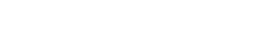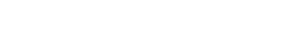A third equilibrium constant can be derived from K1 and KD.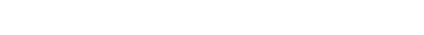The species H2CrO4 and HCr2O7- are only formed at very low pH so they do not appear on this diagram. Published values for log K1 and log KD are 5.89 and 2.05, respectively. Using these values and the equality conditions, the concentrations of the three species, chromate, CrO42-, hydrogen chromate, HCrO4- and dichromate, Cr2O72- can be calculated, for various values of pH, by means of the equilibrium expressions. The chromium concentration is calculated as the sum of the species' concentrations in terms of chromium content.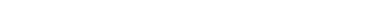The three species all have concentrations equal to 1/KD at pH = pK1, for which [Cr] = 4/KD. The three lines on this diagram meet at that point.
1. Green line. Chromate and hydrogen chromate have equal concentrations. Setting [CrO42-] equal to [HCrO4-] in eq. 1, [H+]=1/K1, or pH = log K1. This relationship is independent of pCr, so it requires a vertical line to be drawn on the predominance diagram.
2. Red line. Hydrogen chromate and dichromate have equal concentrations. Setting [HCrO4-] equal to [Cr2O72-] in Eq. 2, [HCrO4-] = 1/KD; from Eq. 1, then, [CrO42-] = 1/K1[H+].
3. Blue line. Chromate and dichromate have equal concentrations. Setting [CrO42-] equal to [Cr2O72-] in Eq. 3 gives [CrO42-] = 1/β2[H]2.

The predominance diagram is interpreted as follows. The chromate ion is the predominant species in the region to the right of the green and blue lines. Above pH ca. 6.75 it is always the predominant species. At pH < 5.89 (pHK1) the hydrogen chromate ion is predominant in dilute solution but the dichromate ion is predominant in more concentrated solutions.

Predominance diagrams can become very complicated when many polymeric species can be formed as, for example, with vanadate
In chemistry, a vanadate is a compound containing an oxoanion of vanadium generally in its highest oxidation state of +5. The simplest vanadate ion is the tetrahedral, orthovanadate, VO43− anion, which is present in e.g. sodium orthovanadate and in solutions of V2O5 in strong base...

, molybdate
Molybdate
In chemistry a molybdate is a compound containing an oxoanion with molybdenum in its highest oxidation state of 6. Molybdenum can form a very large range of such oxoanions which can be discrete structures or polymeric extended structures, although the latter are only found in the solid state.The...

and tungstate
Tungstate
In chemistry a tungstate is a compound that contains an oxoanion of tungsten or is a mixed oxide containing tungsten. The simplest tungstate ion is WO42−, "orthotungstate"...

. Another complication is that many of the higher polymers are formed extremely slowly, such that equilibrium may not be attained even in months, leading to possible errors in the equilibrium constants and the predominance diagram.